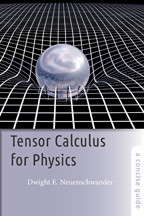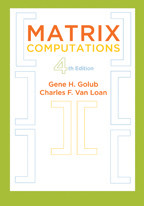Back to Results# Tensor Calculus for Physics

A Concise Guide

Dwight E. Neuenschwander

Publication Date
Request Exam CopyRequest Review Copy

Using a clear, step-by-step approach, this book explains one of the more difficult—yet crucial—topics in physics.

Understanding tensors is essential for any physics student dealing with phenomena where causes and effects have different directions. A horizontal electric field producing vertical polarization in dielectrics; an unbalanced car wheel wobbling in the vertical plane while spinning about a horizontal axis; an electrostatic field on Earth observed to be a magnetic field by orbiting astronauts—these are some situations where physicists employ tensors. But the true beauty of tensors lies…

Using a clear, step-by-step approach, this book explains one of the more difficult—yet crucial—topics in physics.

Understanding tensors is essential for any physics student dealing with phenomena where causes and effects have different directions. A horizontal electric field producing vertical polarization in dielectrics; an unbalanced car wheel wobbling in the vertical plane while spinning about a horizontal axis; an electrostatic field on Earth observed to be a magnetic field by orbiting astronauts—these are some situations where physicists employ tensors. But the true beauty of tensors lies in this fact: When coordinates are transformed from one system to another, tensors change according to the same rules as the coordinates. Tensors, therefore, allow for the convenience of coordinates while also transcending them. This makes tensors the gold standard for expressing physical relationships in physics and geometry.

Undergraduate physics majors are typically introduced to tensors in special-case applications. For example, in a classical mechanics course, they meet the "inertia tensor," and in electricity and magnetism, they encounter the "polarization tensor." However, this piecemeal approach can set students up for misconceptions when they have to learn about tensors in more advanced physics and mathematics studies (e.g., while enrolled in a graduate-level general relativity course or when studying non-Euclidean geometries in a higher mathematics class).

Dwight E. Neuenschwander's Tensor Calculus for Physics is a bottom-up approach that emphasizes motivations before providing definitions. Using a clear, step-by-step approach, the book strives to embed the logic of tensors in contexts that demonstrate why that logic is worth pursuing. It is an ideal companion for courses such as mathematical methods of physics, classical mechanics, electricity and magnetism, and relativity.

Tensor Calculus for Physics

Dwight E. Neuenschwander

Publication Date
Request Exam CopyRequest Review Copy
Related

## Related BooksMatrix Computations

Gene H. Golub and Charles F. Van Loan

fourth edition
\$76.00
Matrix Computations

Gene H. Golub and Charles F. Van Loan

fourth edition
Publication DateQuantum Steampunk

Nicole Yunger Halpern

\$29.95
Quantum Steampunk

Nicole Yunger Halpern

Publication DateTime Travel

Brian Clegg

\$14.95
Time Travel

Brian Clegg

Publication DateRenewable Energy

Stephen Peake

\$14.95
Renewable Energy

Stephen Peake

Publication DateAstrobiology

Kevin W. Plaxco and Michael Gross

third edition
\$34.95
Astrobiology

Kevin W. Plaxco and Michael Gross

third edition
Publication Date
Reviews

## Reviews

This book is well written and has sufficient rigor to allow students to use it for independent study.

An introductory Tensor Calculus for Physics book is a most welcome addition... Professor Neuenschwander's book fills the gap in robust fashion.

## Book Details

Publication Date
Status
Available
Trim Size
6
x
9
Pages
248
ISBN
9781421415659
Illustration Description
8 line drawings
Subject

Preface
Acknowledgments
Chapter 1. Tensors Need Context
1.1. Why Aren't Tensors Defined by What They Are?
1.2. Euclidean Vectors, without Coordinates
1.3. Derivatives of Euclidean Vectors with Respect to a

Preface
Acknowledgments
Chapter 1. Tensors Need Context
1.1. Why Aren't Tensors Defined by What They Are?
1.2. Euclidean Vectors, without Coordinates
1.3. Derivatives of Euclidean Vectors with Respect to a Scalar
1.4. The Euclidean Gradient
1.5. Euclidean Vectors, with Coordinates
1.6. Euclidean Vector Operations with and without Coordinates
1.7. Transformation Coefficients as Partial Derivatives
1.8. What Is a Theory of Relativity?
1.9. Vectors Represented as Matrices
1.10. Discussion Questions and Exercises
Chapter 2. Two-Index Tensors
2.1. The Electric Susceptibility Tensor
2.2. The Inertia Tensor
2.3. The Electric Quadrupole Tensor
2.4. The Electromagnetic Stress Tensor
2.5. Transformations of Two-Index Tensors
2.6. Finding Eigenvectors and Eigenvalues
2.7. Two-Index Tensor Components as Products of Vector Components
2.8. More Than Two Indices
2.9. Integration Measures and Tensor Densities
2.10. Discussion Questions and Exercises
Chapter 3. The Metric Tensor
3.1. The Distinction between Distance and Coordinate Displacement
3.2. Relative Motion
3.3. Upper and Lower Indices
3.4. Converting between Vectors and Duals
3.5. Contravariant, Covariant, and "Ordinary" Vectors
3.6. Tensor Algebra
3.7. Tensor Densities Revisited
3.8. Discussion Questions and Exercises
Chapter 4. Derivatives of Tensors
4.1. Signs of Trouble
4.2. The Affine Connection
4.3. The Newtonian Limit
4.4. Transformation of the Affine Connection
4.5. The Covariant Derivative
4.6. Relation of the Affine Connection to the Metric Tensor
4.7. Divergence, Curl, and Laplacian with Covariant Derivatives
4.8. Disccussion Questions and Exercises
Chapter 5. Curvature
5.1. What Is Curvature?
5.2. The Riemann Tensor
5.3. Measuring Curvature
5.4. Linearity in the Second Derivative
5.5. Discussion Questions and Exercises
Chapter 6. Covariance Applications
6.1. Covariant Electrodynamics
6.2. General Covariance and Gravitation
6.3. Discussion Questions and Exercises
Chapter 7. Tensors and Manifolds
7.1. Tangent Spaces, Charts, and Manifolds
7.2. Metrics on Manifolds and Their Tangent Spaces
7.3. Dual Basis Vectors
7.4. Derivatives of Basis Vectors and the Affine Connection
7.5. Discussion Questions and Exercises
Chapter 8. Getting Acquainted with Differential Forms
8.1. Tensors as Multilinear Forms
8.2. 1-Forms and Their Extensions
8.3. Exterior Products and Differential Forms
8.4. The Exterior Derivative
8.5. An Application to Physics: Maxwell's Equations
8.6. Integrals of Differential Forms
8.7. Discussion Questions and Exercises
Appendix A: Common Coordinate Systems
Appendix B: Theorem of Alternatives
Appendix C: Abstract Vector Spaces
Bibliography
Index

Author Bio
Featured Contributor

## Dwight E. Neuenschwander, Ph.D.

Dwight E. Neuenschwander is a professor of physics at Southern Nazarene University. He is a columnist for the Observer, the magazine of the Society for Physics Students, and the author of Emmy Noether's Wonderful Theorem, also published by Johns Hopkins, and How to Involve Undergraduates in Research: A Field Guide for Faculty.